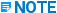# Retrieving and Reporting Bolt Data - PDS - Help

## Plant Design System (PDS) Report Manager (PD_Report)

PPMProduct
PDS
PPMCategory_custom
Help

The system performs the following actions to determine the bolt data to report. Some of these steps are explained in more detail in the following sections.

• Scans the model for two connected components.

• Checks the end preparations of the components to see if the components are bolted.

• Determines the following values for components A and B based on the TDB data. (Component A is the first mating component found when scanning the model.)

• bolt_type_option_code - option code for bolt type (default is 1)

• Piping Materials Class

• bolt item name - this value is set in the RDB.

• maximum temperature - this value is the largest of the four temperature values (Operating and Design) defined in the segment entities.

• NPD of the connected components

• End preparation of connected component A (0 indicates that the spec has no end prep defined)

• pressure rating of component A

• pressure rating of component B

• Calls a Physical Dimension table which returns the number of bolts, the diameter, and the bolt extension.

The table used for bolt reporting is determined by the Length Calculation option on the Material Takeoff Options form in the Project Administrator.

If the Length Calculation field is set to Almost Precise, a table name using the pressure rating and table suffix at the connect point is used, for example, STUD_150_5.

If the Length Calculation field is set to Precise, a table name using the termination type, pressure rating and table suffix at the connect point is used, for example, STUD_20_150_5.

Both tables return the bolt diameter, the number of bolts per mating for the nominal diameter at the connect point, and a set of bolt extensions for the applicable bolt diameter. This also causes lap joint flanges to calculate bolts specially.For more information on length calculations, see Almost Precise and Precise Length Calculation / Bolt Reporting.

• Compares the returned values for components A and B. If the values for the number or bolts, bolt diameter, or bolt circle diameter differ, the system writes an error to the report error log and does not report on that set of bolts.

• For regular bolts, the system accumulates the bolt length as:

• the extension of the bolt at A

• the thickness of flange A

• the gasket separation between the mating flanges A and B

• the thickness of flange B

• the extension of the bolt at B

• and some roundoff factor for preferred bolt lengths.

• For bolt-thru items, the system accumulates the bolt length as:

• the extension of the bolt at A

• the thickness of flange A

• the gasket separation between flange A and the bolt-thru item

• the thickness of the bolt-thru item

• the gasket separation between the bolt-thru item and flange B

• the thickness of flange B

• the extension of the bolt at B

• and some roundoff factor for preferred bolt lengths.

• If more than one bolt-thru item is placed between components A and B, the system calculates the thickness of the item and the gasket separation between each bolt-thru item.

• The bolt length varies with the entry for modifier attribute in the Piping Commodity Specification Data Table (pdtable_202). If the attribute is 0 or positive, it is a stud bolt which has two bolt extensions, and if the attribute is set to negative, it is a machine bolt which has one bolt extensions. For machine bolts, the system uses the larger of the two bolt extensions. This extension includes the thickness of the nut and the total length of the visible threads.

• The system retrieves one-half the gasket separation for each component from the Design Database (dd_projname) and multiplies this value by 2 to get the full gasket gap size. Then it compares the gasket gap at A and B and uses the larger of the two values.

• Uses the information obtained from the TDB defined by the Data, Revise, Revise Bolt Option command sequence in Piping Design Graphics, which sets the bolt override in graphics, to scan the Piping Job Specification for the first unique entry formed by the search criteria.